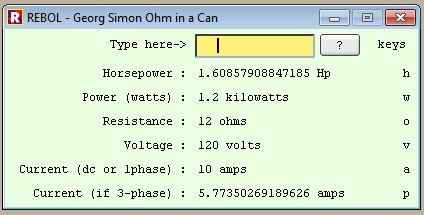Neocities / Hodgepodge / Programming / Rebol Ohms
```
(this is just a screenshot; code is below that)The code (ohm.r):

rebol[title: "Georg Simon Ohm in a Can"]

; still has a bug when calculating r to be infinite ohms
; it'll show it as zero ohms

r: none ; resistance
i: none ; current
v: none ; voltage
p: none ; power in watts
hp: none ; horsepower
iph: none ; amps per phase

;fallback: func[a b][unless attempt a [error-response  b] ]

to-number: func[s /local n][
either attempt [n: to-decimal s][n]
]

HP_to_W: does [p: hp * 746]
W_to_HP: does [hp: p / 746]
IPH_to_I: does [i: iph * (square-root 3)]
I_to_IPH: does [iph: i / (square-root 3)]

calcVI: does [ unless attempt [ r: v / i   p: v * i ] [r: 0  p: 0] ]
calcVR: does [ unless attempt [ i: v / r   p: v * i ] [i: 0  p: 0] ]
calcVP: does [ unless attempt [ i: p / v   r: v / i ] [i: 0  r: 0] ]
calcIR: does [ v: i * r   p: v * i ]
calcIP: does [ unless attempt [ v: p / i   r: v / i ] [v: 0  r: 0] ]
calcRP: does [
unless attempt [
v: square-root(p * r)  i: square-root(p / r)
][
v: 0  i: 0
]
]

calc: does[
if hp[HP_to_W]
if iph[IPH_to_I]  ; get watts or DC amps
case[
all[v i][calcVI]
all[v r][calcVR]
all[v p][calcVP]
all[i r][calcIR]
all[i p][calcIP]
all[r p][calcRP]
true[exit] ; need TWO values to calc so don't do what's below
]
W_to_HP
I_to_IPH  ; set horsepower and amps/phase
]

metricVals: ["m" 1E-3  "u" 1E-6  "n" 1E-9  "" 1  "k" 1E+3  "M" 1E+6  "g" 1E+9]

suf2plain: func[n /local s][
unless attempt[s: to-string last n][return 0]
either(find "munkMg" s)[
n: to-number copy/part n (length? n) - 1
return n * select/case metricVals s
][
]
]

plain2suf: func[n /local a s /ohms][
if n = none [return ""]
a: abs n
case[
a = 0    [s: " "]
a < 1E-6 [n: n * 1E9  s: " nano"]
a < 1E-3 [n: n * 1E6  s: " micro"]
a < 1    [n: n * 1E3  s: " milli"]
a >= 1E9 [n: n / 1E9  s: " giga"]
a >= 1E6 [n: n / 1E6  s: either ohms[" meg"][" mega"]]
a >= 1E3 [n: n / 1E3  s: either ohms["k "][" kilo"]]
true     [s: " "]
]
if n = to-integer n [n: to-integer n] ; changes type, leaves same value
return join (to-string n) s
]

pr: func[c s][c/text: to-string s  show c] ; convenience setTtext

e: does[suf2plain ent/text] ; what you just entered, with suffix processed

fillForm: does[
pr lhp   either(hp = none)[""][join (plain2suf hp) "Hp"]
pr lwatt either(p = none)[""][join (plain2suf p)   "watts"]
pr lohm  either(r = none)[""][join (plain2suf/ohms r)   "ohms"]
pr lvolt either(v = none)[""][join (plain2suf v)   "volts"]
pr lamp  either(i = none)[""][join (plain2suf i)   "amps"]
pr lcpp  either(iph = none)[""][join (plain2suf iph) "amps"]
pr ent ""
] ; right. It fills out the form.

cfc: does [calc fillForm clr]

keyW: does[p: e  cfc]
keyV: does[v: e  cfc]
keyR: does[r: e  cfc]
keyI: does[i: e  cfc]
keyHP: does[hp: e  cfc]
keyIPH: does[iph: e  cfc]

clr: does[
if any[all[r i] all[r v] all[r p] all[i v] all[i p] all[v p]][r: i: v: p: hp: iph: none]
] ; resets'em all to none, IF calc has been done

clrF: does[
r: i: v: p: hp: iph: none
pr lhp ""  pr lwatt ""  pr lohm ""
pr lvolt ""  pr lamp ""  pr lcpp ""
]

bkc: 235.255.225

helpbox: layout[
tt "Type into the only text-entry field it has."
tt "type a number, which can contain single-letter suffixes:"
tt "m(=milli), k(=kilo), u(=micro),"
tt "M(=mega), g(=giga), n(=nano) ."
tt "End the field with one of the ohm's-laws units:"
tt "h(=horsepower), o(=ohms), a(=amps, DC or singlephase),"
tt "v(=volts), w(=watts), p(=amps/phase on 3-phase line)"
]

h: does[view/new center-face helpbox]

view/new layout[
style ttg tt right
style ttr tt 180 right
style ttp tt 200
origin 5x5 space 5x5
across
backdrop bkc
ttr "Type here->" ent: field 120
btn 40 "?" keycode[#"?"][h]
lkt: ttg 44 "keys" keycode[#"^["][clrF] return

ttr "Horsepower :"             lhp:   ttp  lk8: ttg "h" keycode[#"h"][keyHP] return
ttr "Power (watts) :"          lwatt: ttp  lk7: ttg "w" keycode[#"w"][keyW] return
ttr "Resistance :"             lohm:  ttp  lk6: ttg "o" keycode[#"o"][keyR] return
ttr "Voltage :"                lvolt: ttp  lk5: ttg "v" keycode[#"v"][keyV] return
ttr "Current (dc or 1phase) :" lamp:  ttp  lk4: ttg "a" keycode[#"a"][keyI] return
ttr "Current (if 3-phase) :"   lcpp:  ttp  lk3: ttg "p" keycode[#"p"][keyIPH]
]

focus ent do-events quit

```